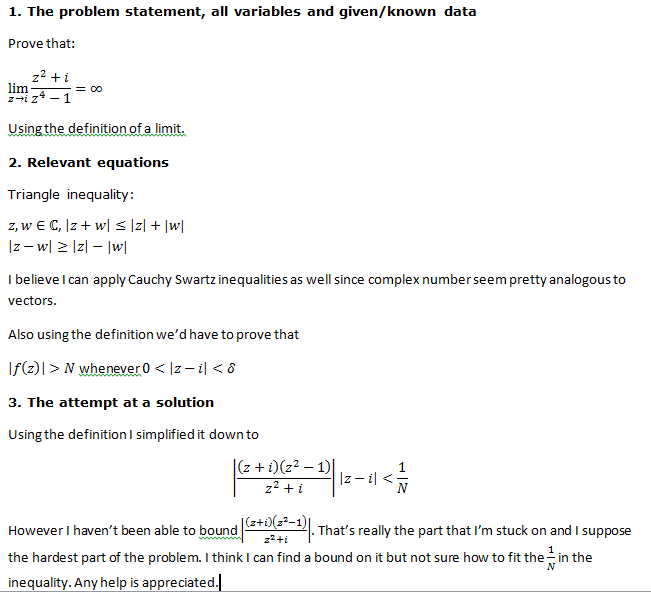# Prove lim z->i ((z^2+i)/(z^4-1))=∞

thed0ctorStaff Emeritus
Homework Helper
Gold Member
Please, Give us something readable without us having to switch back & forth between tabs/windows.Homework Helper
Gold Member
Instead of trying to bound the expression above by 1/N, why not apply what you know about the algebra of limits?

$$\lim_{z \rightarrow i} \frac{(z + i)(z^2 - 1)(z - i)}{z^2 + i} = \frac{[\lim_{z \rightarrow i} (z + i)] [\lim_{z \rightarrow i} (z^2 - 1)][\lim_{z \rightarrow i} (z - i)]}{\lim_{z \rightarrow i} (z^2 + i)}$$

This is valid provided that the limit in the denominator is nonzero.

If you can prove that the above limit is zero, then that implies your 1/N bound and you can proceed accordingly.

Staff Emeritus
Homework Helper
Gold Member
thed0ctor,

See what bound $\displaystyle \left|z-i\right|<\frac{1}{2}$ puts on $\displaystyle \left|\frac{(z + i)(z^2 - 1)}{z^2 + i}\right|\ .$

thed0ctor
Instead of trying to bound the expression above by 1/N, why not apply what you know about the algebra of limits?

$$\lim_{z \rightarrow i} \frac{(z + i)(z^2 - 1)(z - i)}{z^2 + i} = \frac{[\lim_{z \rightarrow i} (z + i)] [\lim_{z \rightarrow i} (z^2 - 1)][\lim_{z \rightarrow i} (z - i)]}{\lim_{z \rightarrow i} (z^2 + i)}$$

This is valid provided that the limit in the denominator is nonzero.

If you can prove that the above limit is zero, then that implies your 1/N bound and you can proceed accordingly.

I wasn't 100% sure of how proving that limit equals zero would help but I did take your advice and broke the problem into several limits whose products are infinity.

thed0ctor,

See what bound $\displaystyle \left|z-i\right|<\frac{1}{2}$ puts on $\displaystyle \left|\frac{(z + i)(z^2 - 1)}{z^2 + i}\right|\ .$

I used the idea of bounding |z+i| by 3 by assuming delta was at most 1. I attached a pdf of my work. If someone could check it to make sure my logic is alright I'd be very grateful. I've just recently found out how epsilon and deltas work.

#### Attachments

• problem limit.pdf
1.1 MB · Views: 295
Staff Emeritus
Homework Helper
Gold Member
...
I used the idea of bounding |z+i| by 3 by assuming delta was at most 1. I attached a pdf of my work. If someone could check it to make sure my logic is alright I'd be very grateful. I've just recently found out how epsilon and deltas work.
$\displaystyle \frac{-1+i}{\sqrt{2}}$ is less that 1 unit from i on the complex plane, so δ = 1 is too large.

$\displaystyle \left(\frac{-1+i}{\sqrt{2}}\right)^2=-i$ giving division by zero for the denominator of z2 + i .

thed0ctor
$\displaystyle \frac{-1+i}{\sqrt{2}}$ is less that 1 unit from i on the complex plane, so δ = 1 is too large.

$\displaystyle \left(\frac{-1+i}{\sqrt{2}}\right)^2=-i$ giving division by zero for the denominator of z2 + i .

Sorry the last bit was meant to say

$$∴ \lim_{z \rightarrow i} \frac{z^2 + i}{z^4 -1} = ∞$$
not:
$$∴ \lim_{z \rightarrow i} \frac{z^2 + i}{z^4 -i} = ∞$$

Does this fix that issue? Because now I don't see the division by zero issue.

Staff Emeritus
Homework Helper
Gold Member
Sorry the last bit was meant to say

$$∴ \lim_{z \rightarrow i} \frac{z^2 + i}{z^4 -1} = ∞$$
not:
$$∴ \lim_{z \rightarrow i} \frac{z^2 + i}{z^4 -i} = ∞$$

Does this fix that issue? Because now I don't see the division by zero issue.
That depends ...

Is it acceptable to split-up the limit as you did in the PDF file? If so, then the proof at the end is fine.

However, if you are to use the definition for the given expression, then your first attempt was a good start. The algebra may be pretty involved to find what the bound is, depending on what restriction you place on δ.

thed0ctor
That depends ...

Is it acceptable to split-up the limit as you did in the PDF file? If so, then the proof at the end is fine.

However, if you are to use the definition for the given expression, then your first attempt was a good start. The algebra may be pretty involved to find what the bound is, depending on what restriction you place on δ.

Good point. I also believe I found a bound. it's a nasty number but it's off the assumption that |z-i|< δ< .5

I attached the pdf. I think I'm getting better with inequalities. Thanks so much for your help thus far!

#### Attachments

• bounding.pdf
1.1 MB · Views: 198
Homework Helper
Gold Member
I just skimmed through your PDF and I think I found a problem.

You established that $|z^2 + i| < 11/2$ but then you applied this with $|z^2 + i|$ in the denominator. In that case, you need to reverse the inequality:

$$\frac{1}{|z^2 + i|} > \frac{1}{11/2}$$
Therefore your final inequality is not correct.

If you want an upper bound for $1/|z^2 + i|$, then you need a LOWER bound for $|z^2 + i|$.

Homework Helper
Gold Member
By the way, here's what I was getting at earlier in message #3. Suppose you have proved that

$$\lim_{z \rightarrow i} \frac{z^4 - 1}{z^2 + i} = 0$$
Then this means, by definition, that given N > 0 there exists $\delta > 0$ such that
$$\left| \frac{z^4 - 1}{z^2 + i} \right| < \frac{1}{N}$$
whenever $0 < |z - i| < \delta$. But that's exactly what you need, right? (Just take the reciprocal of the above inequality.) Therefore the problem reduces to showing that
$$\lim_{z \rightarrow i} \frac{z^4 - 1}{z^2 + i} = 0$$
and you can do this quite simply by using the algebra of limits (i.e., the limit of the quotient is the quotient of the limits), instead of playing around with all these inequalities.

Of course, if your instructor intends for you to do a full delta-epsilon proof for everything, then you should carry on doing that. It's hard to tell from the problem statement.

Last edited: